Simple harmonic motion facts for kids

Kids Encyclopedia Facts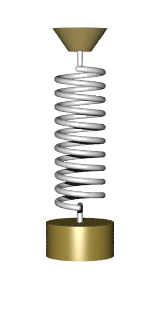A weight on a spring shows simple harmonic motion.

In mathematics and physics, when something moves so that its distance from a fixed point (plotted on a graph against time) looks like a sine wave, the movement is called simple harmonic motion. This sort of movement will happen whenever the force towards the fixed point is proportional to the distance from the point and always acts towards that point. Examples include a weight on a spring and a pendulum (approximately).

The amplitude is the biggest distance from the fixed point. The period is the time taken to get back to the same point again (with the same speed and in the same direction).

The equations of simple harmonic motion can be found by looking at a fixed wheel with radius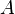$A$ that is spinning with steady speed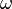$\omega$ radians per second. The time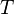$T$ taken for one complete turn is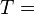$T =$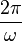$2\pi\over\omega$ because there are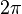$2\pi$ radians in a full circle.

Imagine a white spot painted on the rim of the wheel. If it starts level with the axle, and the wheel has turned through an angle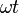$\omega t$ in time$t$ seconds, then the height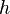$h$ of the spot above the axle is given by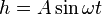$h = A \sin \omega t$ (where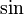$\sin$ means the sine of the angle turned, and trigonometry is used to find the height).

Images for kidsSimple harmonic motion Facts for Kids. Kiddle Encyclopedia.